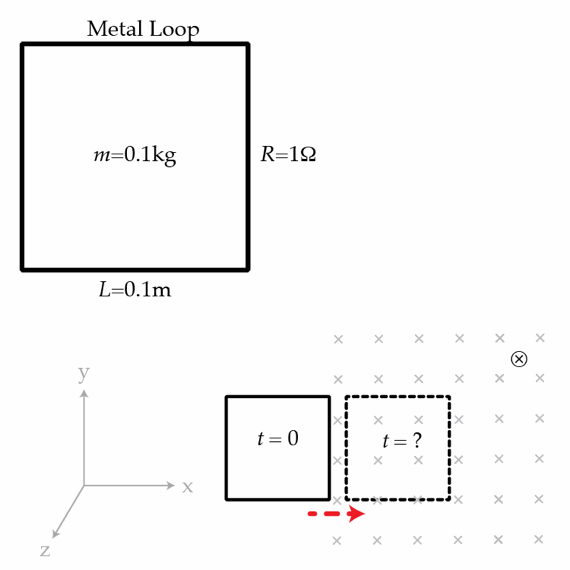# Slow down there, you silly piece of metal

A square loop of metal of side length $L= 0.1~\mbox{m}$, mass $m=0.1~\mbox{kg}$, and resistance $R=1~\Omega$, oriented in the $xy$ plane, is traveling to the right (positive $x$ direction) at a speed of $v_0=0.1~\mbox{m/s}$. At time $t=0$, the right edge of the loop enters a region of magnetic field $B=1\hat{z}~\mbox{T}$. At what time in seconds does the left edge of the loop enter the magnetic field?×

Problem Loading...

Note Loading...

Set Loading...